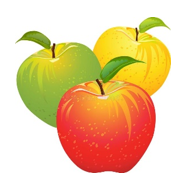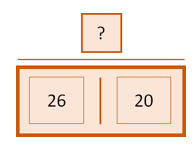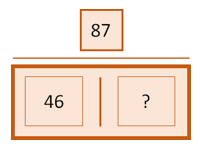Mathematics
Easy

Question

# Farmer Ben picks 87 apples. 26 apples are green, 20 are yellow, and the rest are red. How many apples are red?## The correct answer is: 41

###26 + 20 = ? 26 + 20 = 4687 – 46 = ? 87 – 46 = 41 So, 41 apples are red.

total apple =87
green apple =26
yellow apple =20
lets assume there was 'x' red apple
as we are given that total apple =87
red apple + green apple + yellow apple=87(total apple)
x+26+20=87
x+46=87
x=87-46(we are given rest apple are red in color)
x=41

### Related Questions to study#### With Turito Foundation.#### Get an Expert Advice From Turito.